Difference between revisions of "User:IssaRice/Computability and logic/Diagonalization lemma"

Rogers's fixed point theorem

Let$f$ be a total computable function. Then there exists an index$e$ such that$\varphi_e \simeq \varphi_{f(e)}$.

(simplified)

Define$d(x) = \varphi_x(x)$ (this is actually slightly wrong, but it brings out the analogy better).

Consider the function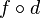$f\circ d$. This is partial recursive, so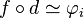$f\circ d \simeq \varphi_i$ for some index$i$.

Now$\varphi_{f(d(i))} \simeq \varphi_{\varphi_i(i)}$ since$f\circ d \simeq \varphi_i$. This is equivalent to$\varphi_{d(i)}$ by definition of$d$. Thus, we may take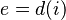$e = d(i)$ to complete the proof.

Diagonalization lemma

(semantic version)

Let$A$ be a formula with one free variable. Then there exists a sentence$G$ such that$G$ iff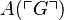$A(\ulcorner G\urcorner)$.

Define$\mathrm{diag}(x)$ to be$\ulcorner C(\ulcorner C\urcorner)\urcorner$ where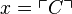$x = \ulcorner C\urcorner$. In other words, given a number$x$, the function$\mathrm{diag}$ finds the formula with that Godel number, then diagonalizes it (i.e. substitutes the Godel number of the formula into the formula itself), then returns the Godel number of the resulting sentence.

Let$B$ be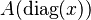$A(\mathrm{diag}(x))$, and let$G$ be$B(\ulcorner B\urcorner)$.

Then$G$ is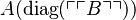$A(\mathrm{diag}(\ulcorner \ulcorner B\urcorner\urcorner))$.